# Electromagnetic Induction

Electromagnetic induction refers to the phenomenon where an emf is induced when the magnetic flux linking a conductor changes.

Magnetic Flux is defined as the product of the magnetic flux density and the area normal to the field through which the field is passing. It is a scalar quantity and its S.I. unit is the weber (Wb).

φ = B A

The Weber is defined as the magnetic flux if a flux density of one tesla passes perpendicularly through an area of one square metre.

Example:
A magnetic field of flux density 20 T passes down through a coil of of wire, making an angle of 60° to the plane of the coil as shown. The coil has 500 turns and an area of 25 cm2. Determine:

 (i) the magnetic flux through the coil φ = B A = 20 (sin 60°) 25 x 10-4 = 0.0433 Wb (ii) the flux linkage through the coil Φ = N φ = 500 x 0.0433 = 21.65 Wb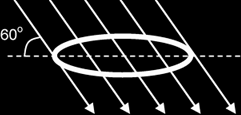Magnetic Flux Linkage is the product of the magnetic flux passing through a coil and the number of turns of the coil.

Φ = N φ = N B A

#### Inducing E.M.F. by changing magnetic flux: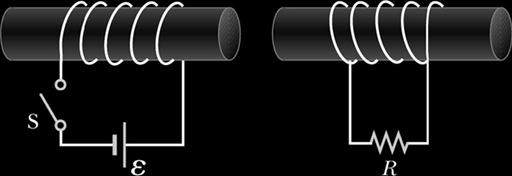In the set up shown above, when the switch S connected to coil A is closed, the galvanometer needle connected to coil B moves to 1 side momentarily.

And when the switch S is opened, the galvanometer needle moves to the other side momentarily.

At the instant when switch S is either opened or closed, there is a change in magnetic flux in coil A.

The movement in the needle of the galvanometer indicates that when there is a change in magnetic flux in coil A, a current passes through coil B momentarily. This suggests that an EMF is generated in coil B momentarily.

#### Factors affecting the magnitude of the induced E.M.F.:

 When a magnet is pushed into a coil as shown, the galvanometer deflects in one direction momentarily.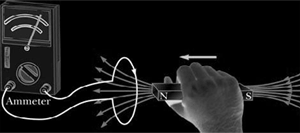When the magnet is not moving, the galvanometer shows no reading.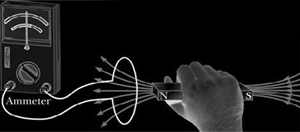When the magnet is withdrawn from the coil, the galvanometer deflects in the opposite direction momentarily.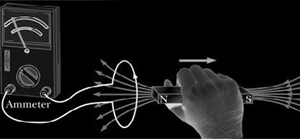When the magnet is moved, its field lines are being “cut” by the coil. This generates an induced EMF in the coil that produces an induced current that flows in the coil, causing the deflection in the ammeter.

The magnitude of the deflection depends on the magnetic field density B, the speed of motion v of the magnet, and the number of turns N in the coil.

The magnitude of induced EMF is directly proportional/equal to the rate of change of magnetic flux-linkage.

|E| = dNBA / dt

Lenz's Law:
The direction of the induced EMF is such that its effects oppose the change which causes it, or The induced current in a closed loop must flow in such a direction that its effects opposes the flux change {or change} that produces it

Example
Explain how Lenz's Law is an example of the law of conservation of energy:
{Illustrate with diagram of a coil “in a complete circuit”, bar magnet held in hand of a person (= external agent)}

• As the ext agent causes the magnet to approach the coil, by Lenz‟s law, a current is induced in such a direction that the coil repels the approaching magnet.
• Consequently, work has to be done by the external agent to overcome this opposition, and
• It is this work done which is the source of the electrical energy {Not: induced emf}

For a straight conductor “cutting across” a B-field: E = B L v sin θ

For a coil rotating in a B-field with angular frequency ω:

E = N B A ω cos ω t, if φ = BAsinωt
& E = N B A ω sin ω t, if φ = BAcosωt

{Whether φ = BAsinωt, or = BAcosωt, would depend on the initial condition}

The induced EMF is the negative of the gradient of the φ ~ t graph {since E = -d / dt}

→ the graphs of E vs t & φ vs t , for the rotating coil have a phase difference of 900.

#### Applications of electromagnetic induction

 Eddy Currents Eddy currents are currents induced in metals moving in a magnetic field or metals that are exposed to a changing magnetic field. Consider a solid metallic cylinder rotating in a B-field as shown: A force resisting the rotation would be generated as shown. Heat would be generated by the induced current in cylinder To reduce eddy currents, the solid cylinder could be replaced with a stack of “coins” with insulation between one another. The insulation between the coins increases resistance and reduces eddy current, thus reducing friction or heating.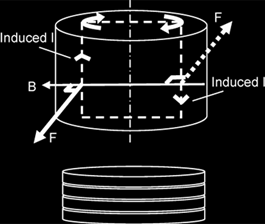Applications of Eddy Currents

1. Induction Cooker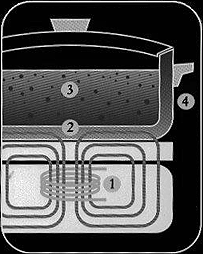Changing magnetic fields in the stove generate eddy currents in the base of the metal pot placed on it, thus producing heat. The element's electronics power a coil that produces a high-frequency electromagnetic field. The field penetrates the metal of the ferrous (magnetic-material) cooking vessel and sets up a circulating eddy current, which generates heat. The heat generated in the cooking vessel is transferred to the vessel's contents. Nothing outside the vessel is affected by the field--as soon as the vessel is removed from the element, or the element turned off, heat generation stops. (Note: the process described at #2 above is called an "eddy current"; in fact, most of the heating is from "hysteresis", which means the resistance of the ferrous material to rapid changes in magnetization--but the general idea is the same: the heat is generated in the cookware).

2. Metal detectors

A pulsing current is applied to the coil, which then induces a magnetic field shown. When the magnetic field of the coil moves across metal, such as the coin in this illustration, the field induces electric currents (called eddy currents) in the coin. The eddy currents induce their own magnetic field, which generates an opposite current in the coil, which induces a signal indicating the presence of metal.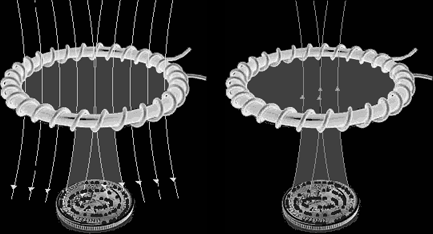3. Electric guitars

Electric guitars use electromagnetic pickups in which an induced emf is generated in a coil of wire by a vibrating string. Most guitars have at least two pickup coils located below each string. Each pickup is sensitive to different harmonics produced by the vibrating string. The string is made from a magnetisable metal, and the pickup consists of a coil of wire within which a permanent magnet is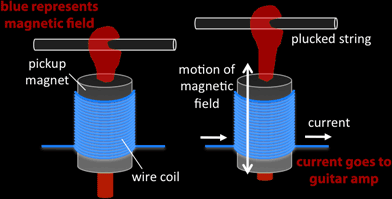located. The permanent magnet produces a magnetic field that penetrates the guitar string, causing it to become magnetized with north and south poles. When the string is plucked, it oscillates, thereby changing the magnetic flux that passes through the coil. The changing magnetic flux induces an emf in the coil, and the polarity of this emf alternates with the vibratory motion of the string. A string vibrating at 440 Hz, for example, induces a 440-hz ac emf in the coil. This signal, after being amplified, is sent to the speakers, which produce a 440-Hz sound wave.

4. Earth-leakage circuit breaker (ELCB)

ELCB is used in electrical circuits in the home to protect the users from electric shocks when there is a fault in the electrical appliance. The ELCB can be triggered to stop a current, depending on whether an induced voltage appears across a sensing coil.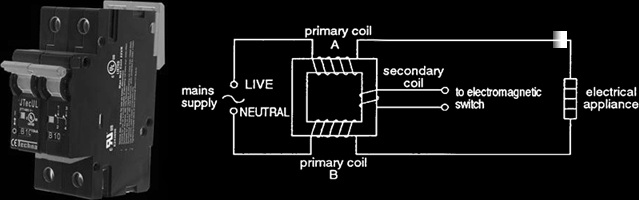The sensing coil is wrapped around an iron ring, through which the current carrying wire passes. Under normal operating conditions, the current flowing in the coils A and B are equal but opposite directions. The magnetic field produced by both coils will always be equal and opposite, hence cancelling out each other at the secondary or sensing coil. The situation changes when there is a leakage in the electrical appliance, where the current returning is smaller than the current going into the appliance. Under this condition, the net magnetic field through the secondary coil is no longer zero and changes with time, since the current is ac. The changing magnetic flux causes an induced voltage to appear in the secondary coil, which triggers the circuit breaker to stop the current. ELCB works very fast (in less than a millisecond) and turn off the current before it reaches a dangerous level.

5. Eddy current brake

An eddy current brake, like a conventional friction brake, is responsible for slowing an object, such as a train or a roller coaster. Unlike friction brakes, which apply pressure on two separate objects, eddy current brakes slow an object by creating eddy currents through electromagnetic induction which create resistance, and in turn either heat or electricity.

Consider a metal disk rotating clockwise through a perpendicular magnetic field but confined to a limited portion of the disk area. (Compare this with the Faraday‟s disk earlier)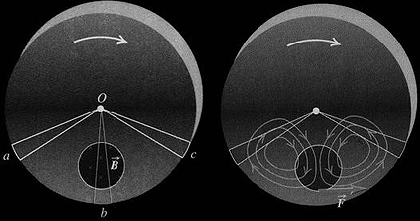Sector Oa and Oc are not in the field, but they provide return conducting path, for charges displaced along Ob to return from b to O. The result is a circulation of eddy current in the disk. The current experiences a magnetic force that opposes the rotation of the disk, so this force must be to the right. The return currents lie outside the field, so they do not experience magnetic forces. The interaction between the eddy currents and the field causes a braking action on the disk.